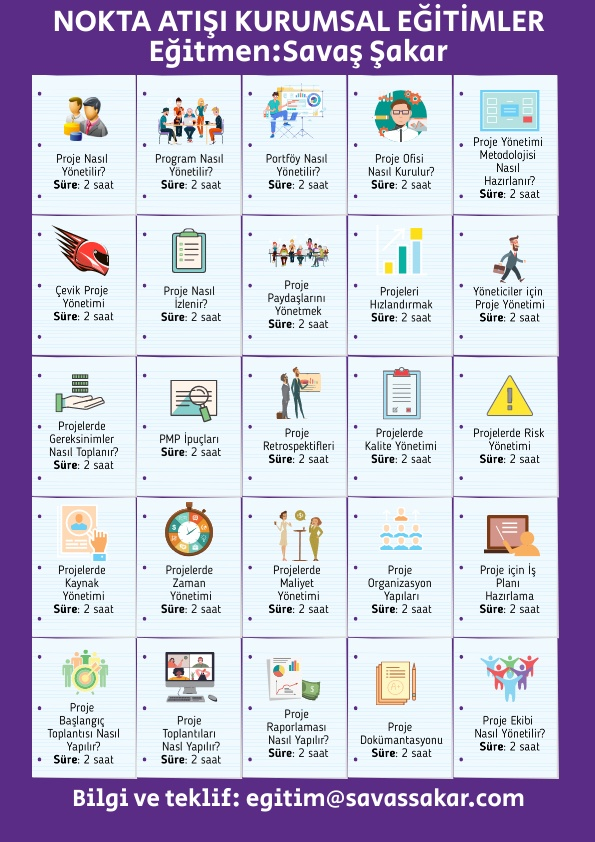# PMP® Formülleri – 2

 1. PERT (P + 4M + O )/ 6 Pessimistic, Most Likely, Optimistic 2. Standard Deviation (P – O) / 6 3. Variance [(P – O)/6 ]squared 4. Float or Slack LS-ES and LF-EF 5. Cost Variance EV – AC 6. Schedule Variance EV – PV 7. Cost Perf. Index EV / AC 8. Sched. Perf. Index EV / PV 9. Est. At Completion (EAC) BAC / CPI, AC + ETC — Initial Estimates are flawed AC + BAC – EV — Future variance are Atypical AC + (BAC – EV) / CPI — Future Variance would be typical 10. Est. To Complete Percentage complete EAC – AC EV/ BAC 11. Var. At Completion BAC – EAC 12. To Complete Performance Index TCPI Values for the TCPI index of less then 1.0 is good because it indicates the efficiency to complete is less than planned. How efficient must the project team be to complete the remaining work with the remaining money? ( BAC – EV ) / ( BAC – AC ) 13. Net Present Value Bigger is better (NPV) 14. Present Value PV FV / (1 + r) 15. Internal Rate of Return Bigger is better (IRR) 16. Benefit Cost Ratio Bigger is better ((BCR or Benefit / Cost) revenue or payback VS. cost) Or PV or Revenue / PV of Cost 17. Payback Period Less is better Net Investment / Avg. Annual cash flow. 18. BCWS PV 19. BCWP EV 20. ACWP AC 21. Order of Magnitude Estimate -25% – +75% (-50 to +100% PMBOK®) 22. Budget Estimate -10% – +25% 23. Definitive Estimate -5% – +10% 24. Comm. Channels N(N -1)/2 25. Expected Monetary Value Probability * Impact 26. Point of Total Assumption (PTA) ((Ceiling Price – Target Price)/buyer’s Share Ratio) + Target Cost Sigma σ 1σ = 68.27% 2σ = 95.45% 3σ = 99.73% Return on Sales ( ROS ) Net Income Before Taxes (NEBT) / Total Sales OR Net Income After Taxes ( NEAT ) / Total Sales Return on Assets( ROA ) NEBT / Total Assets OR NEAT / Total Assets Return on Investment ( ROI ) NEBT / Total Investment OR NEAT / Total Investment Working Capital Current Assets – Current Liabilities Discounted Cash Flow Cash Flow X Discount Factor Contract related formulas Savings = Target Cost – Actual Cost Bonus  =  Savings x Percentage Contract Cost =  Bonus + Fees Total Cost = Actual Cost + Contract Cost
Paylaşın: